Homework Help Question & Answers

# Differential Equations: Please read carefully and choose the best answer 1.1 1.2 1.3 1.4 In the...

Differential Equations: Please read carefully and choose the best answer

1.1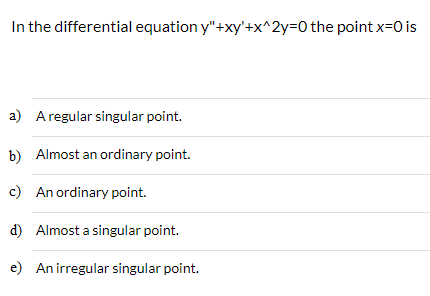1.2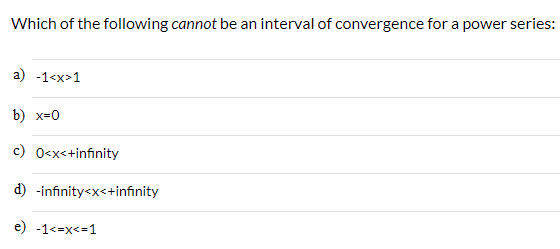1.3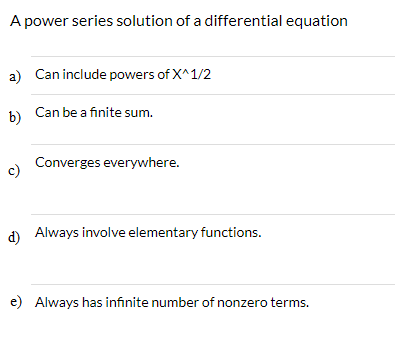1.4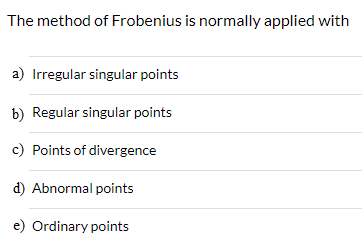In the differential equation y"+xy'+x^2y 0 the point x 0 is a) Aregular singular point. Almost an ordinary point. b) c) An ordinary point. d) Almost a singular point. e) An irregular singular point.
Which of the following cannot be an interval of convergence for a power series: a) -1<x>1 b) x0 c) 0<x<+infinity d) infinity<x<+infinity e) -1<=x<-1
A power series solution of a differential equation Can include powers of X^1/2 a) Can be a finite sum b) Converges everywhere. c) Always involve elementary functions. e) Always has infinite number of nonzero terms.
The method of Frobenius is normally applied with a) Irregular singular points b) Regular singular points c) Points of divergence d Abnormal points e) Ordinary points

#### Homework Answers

Answer #1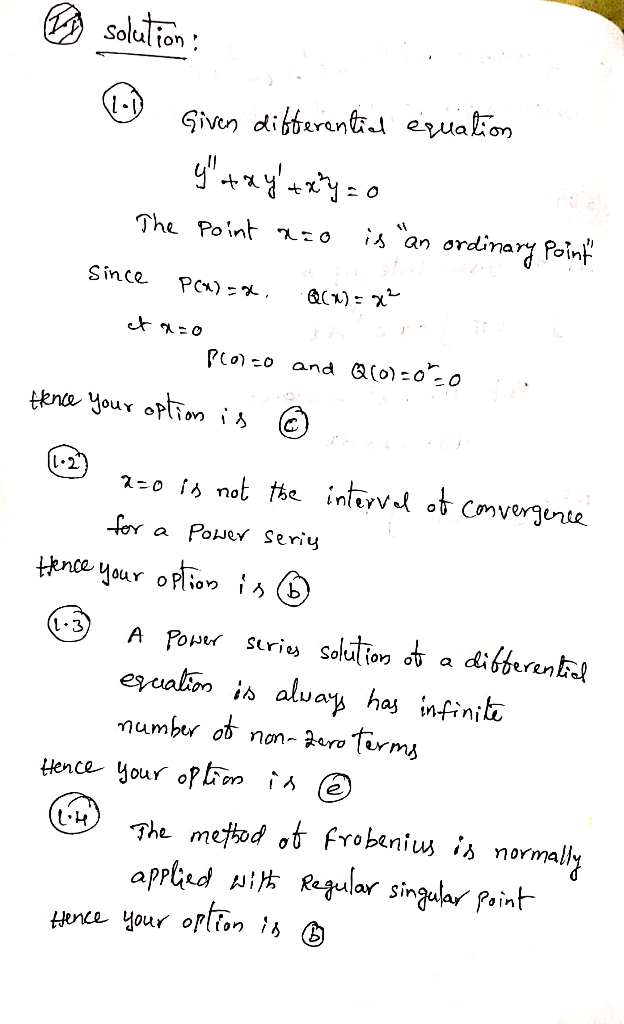Know the answer?
Your Answer:

#### Post as a guest

Your Name:

What's your source?

#### Earn Coin

Coins can be redeemed for fabulous gifts.

Not the answer you're looking for? Ask your own homework help question. Our experts will answer your question WITHIN MINUTES for Free.
Similar Homework Help Questions
• ### Engineering Mathematics IIA Page 3 of 8 3. Consider the second-order ordinary differential equation for y(x)...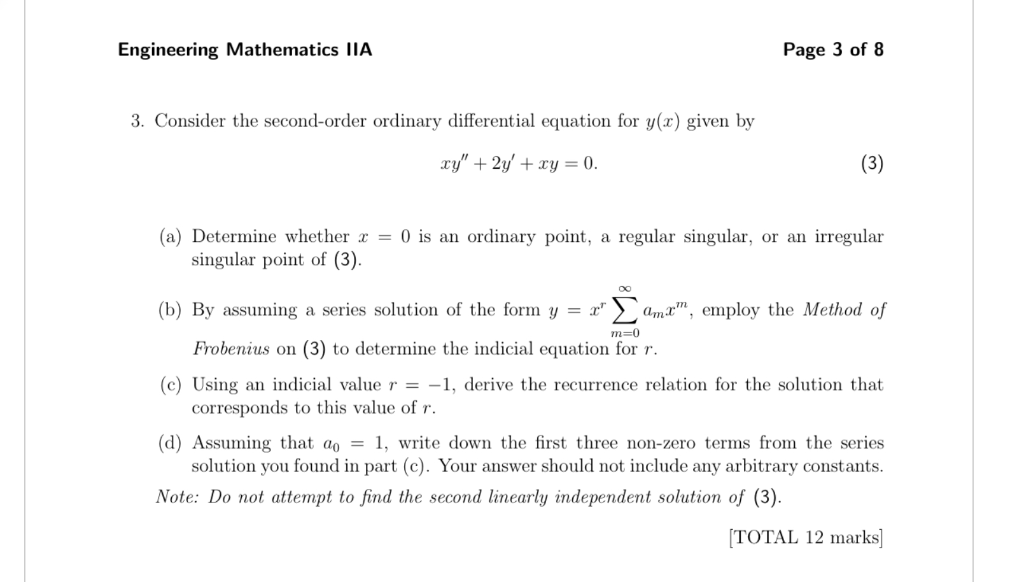Engineering Mathematics IIA Page 3 of 8 3. Consider the second-order ordinary differential equation for y(x) given by (3) xy"2y' +xy = 0. (a) Determine whether = 0 is an ordinary point, regular singular, or an irregular a singular point of (3). (b) By assuming a series solution of the form y = x ama, employ the Method of m-0 Frobenius on (3) to determine the indicial equation for r. (c) Using an indicial value r = -1, derive the...

• ### Consider the differential equation 4x2y′′ − 8x2y′ + (4x2 + 1)y = 0 (a) Verify that x0 = 0 is a regular singular point of...

Consider the differential equation 4x2y′′ − 8x2y′ + (4x2 + 1)y = 0 (a) Verify that x0 = 0 is a regular singular point of the differential equation and then find one solution as a Frobenius series centered at x0 = 0. The indicial equation has a single root with multiplicity two. Therefore the differential equation has only one Frobenius series solution. Write your solution in terms of familiar elementary functions. (b) Use Reduction of Order to find a second...

• ### Find two power series solutions of the given differential equation about the ordinary point x =...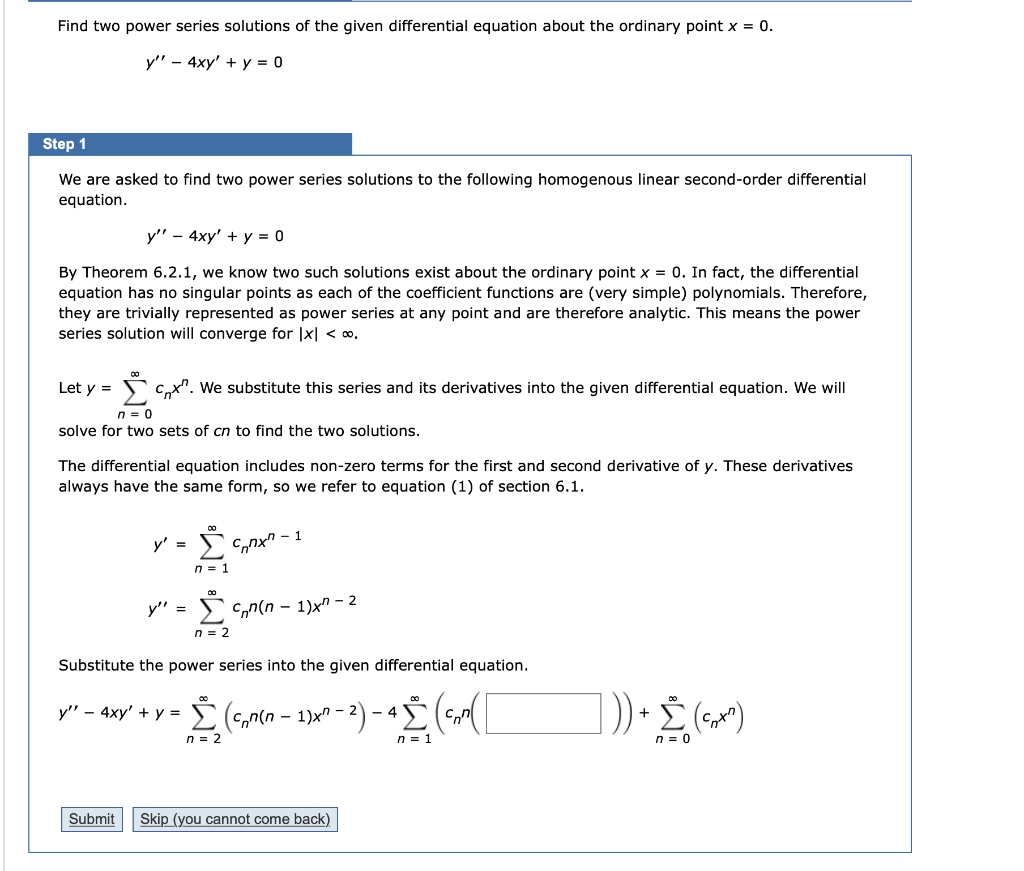Find two power series solutions of the given differential equation about the ordinary point x = 0. y′′ − 4xy′ + y = 0 Find two power series solutions of the given differential equation about the ordinary point x = 0. y!' - 4xy' + y = 0 Step 1 We are asked to find two power series solutions to the following homogenous linear second-order differential equation. y" - 4xy' + y = 0 By Theorem 6.2.1, we know two...

• ### Points) Use the differential equation (x-x- 12 following questions: (a) Find all singular points ...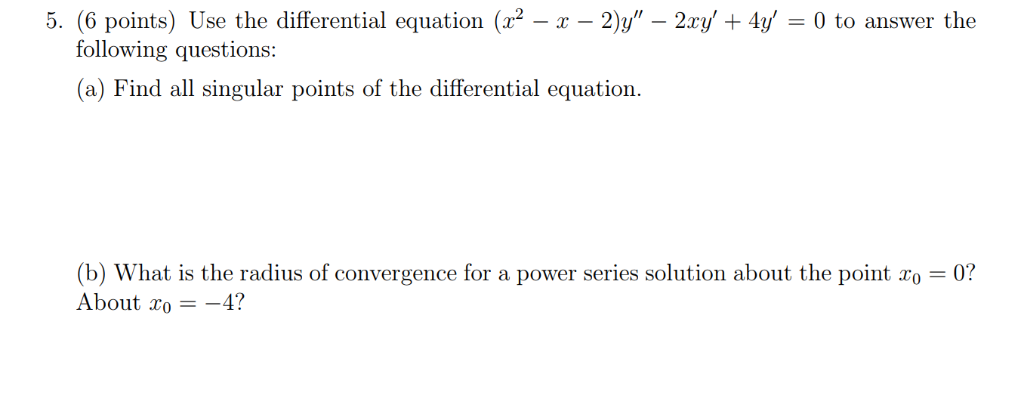points) Use the differential equation (x-x- 12 following questions: (a) Find all singular points of the differential equation. title diferential equation (ra-r-11-2r-w-0 to answer the (b) What is the radius of convergence for a power series solution about the point ro 0? About xo-4? points) Use the differential equation (x-x- 12 following questions: (a) Find all singular points of the differential equation. title diferential equation (ra-r-11-2r-w-0 to answer the (b) What is the radius of convergence for a power series...

• ### Given the DE: y"-(x+1)y'-y=0 use it to answer the following: a) Find the singular point(s), if...

Given the DE: y"-(x+1)y'-y=0 use it to answer the following: a) Find the singular point(s), if any, and if lower bound for the radius of convergence for a power series solution about the ordinary points x=0 b)The recurrence relation Hint: It will be a 3-term recurrence relation c)Give the first four non-zero terms of each of the two linearly independent power series solutions near the ordinary point x=0

• ### Need some help with SERIES SOLUTION - 2nd ORDER EQUATION For the differential equation,          (1) a. Calculate the indicial equation for the power series solution (Answer in a quadratic polynomial...

Need some help with SERIES SOLUTION - 2nd ORDER EQUATION For the differential equation,          (1) a. Calculate the indicial equation for the power series solution (Answer in a quadratic polynomial in terms of c.) b. Calculate the solutions of the indicial equation found above. c. Calculate the point from the above equation (1) as i. ORDINARY POINT ii. REGULAR SINGULAR POINT iii. IRREGULAR SINGULAR POINT We were unable to transcribe this imagey-Σ@m(z _ 4)nte We were unable to transcribe this...

• ### For differential equations please show all parts. For part a) An appropriate method from chapter ...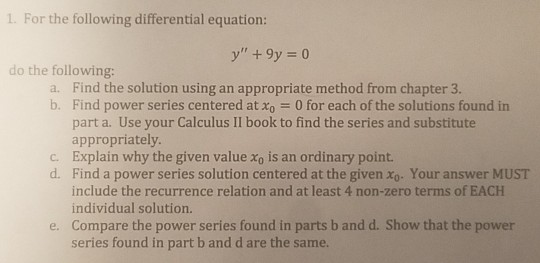For differential equations please show all parts. For part a) An appropriate method from chapter 3 identifies the characteristic equation r^2 +9=0 where r =(+-)3i Thus y=c1cos(3x)+c2sin(3x) So I need help with the remaining parts L. For the following differential equation do the following: a. Find the solution using an appropriate method from chapter 3. b. Find power series centered at x 0 for each of the solutions found in part a. Use your Calculus II book to find the...

• ### Problem 4. (40 points) Consider the differential equation where λ is a positive real constant and...Problem 4. (40 points) Consider the differential equation where λ is a positive real constant and y is a function of x. (a) Is it possible to solve the above differential equation using power series method around ro 0? Explain why? (b) Is it possible to solve the above differential equation using Frobenius method around 20 = 0? Explain why? (c) Write the above differential equation in a proper form for Frobenius method by using the change of variable t...

• ### Consider the following differential equation Note: For each part below you must give your answers in terms of fract...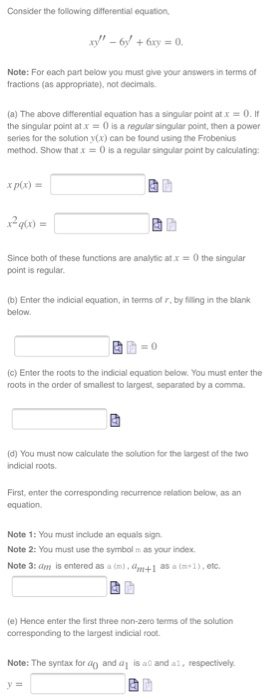Consider the following differential equation Note: For each part below you must give your answers in terms of fractions (as appropriate), not decimals (a) The above differential equation has a snaar point at x 0 . It the singular point at x-0 is a regular singular point, then a power series for the solution y(x) can be lound using the Frobenius method. Show that x = 0 is a regular sigar point by calculating: xp(x) = y(x) = Since both...

• ### Find all singular points of the following equation and determine whether each one is regular or...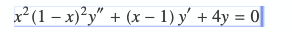Find all singular points of the following equation and determine whether each one is regular or irregular? a) 0 and 1 are regular singular points. b) 1 is a regular singular point, 0 is an irregular singular point. c) 0 is a regular singular point, 1 is an irregular singular point. d) none of these e) 0 and 1 are irregular singular points. x? (1 – x)2y" + (x - 1) y' + 4y = 0

Free Homework App

Scan Your Homework
to Get Instant Free Answers
Need Online Homework Help?

Get Answers For Free
Most questions answered within 3 hours.